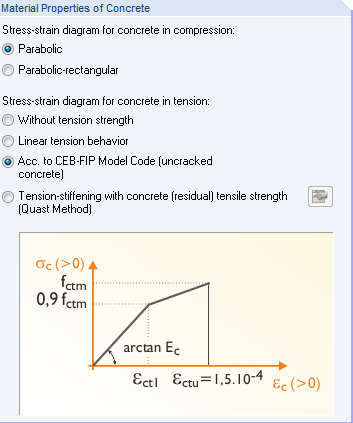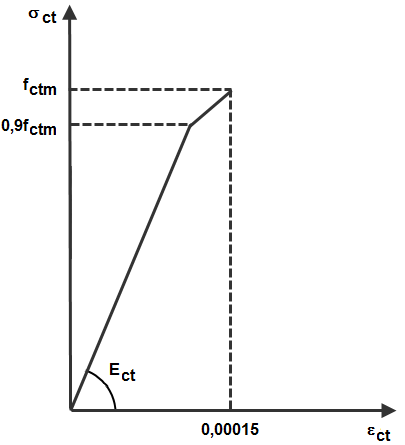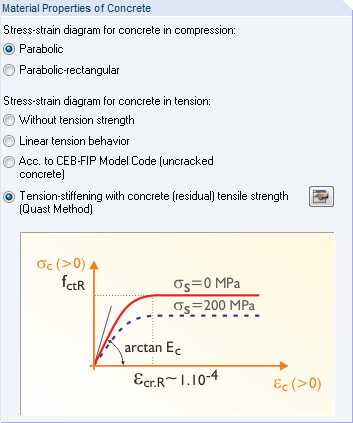# RF-CONCRETE Surfaces – Online Manual Version 5

Online manuals, introductory examples, tutorials, and other documentation.

# 2.8.3.2 Concrete in tension area

#### Concrete in tension area

There are several options available for the stress-strain diagram of the concrete in the tension area.Figure 2.133 Stress-strain diagram for tension area of concrete

The concrete tensile strength can, inter alia, be considered according to CEB-FIP Model Code 90:1993. Until the tensile strength of concrete fctm is reached, the program assumes the distribution shown in Figure 2.134 for reaching the crack strain of 0.15 ‰.Figure 2.134 Stress-strain relation for concrete in the tension area as per CEB-FIP Model Code 90:1993

Alternatively, you can use the concrete's stiffening effect in the tension zone (tension stiffening). This procedure is described in the following chapter.Figure 2.135 Stress-strain diagram for Tension-stiffening